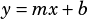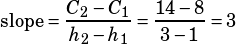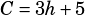Test 1 Calculator OK #16

The graph here is definitely a linear relationship between h and C, so we’re going to want to figure out the slope and the C-intercept. Then we’ll use the standardslope-intercept formula, only for this problem it’ll be.

Slope is rise over run, or the change in the vertical variable divided by the change in the horizontal variable. Let’s pick two points from the line and plug them into the slope formula. How about (1, 8) and (3, 14)?Once we have the slope, we’re actually done because whoever wrote this question wasn’t very tricky with the answer choices. The C-intercept of this graph is obviously not zero, and only choice C has the slope we just calculated plus a non-zero C-intercept. Sure enough, a quick glance at the graph confirms that the C-intercept is 5, meaning the equation should be.